# Ideal Points and Vanishing Points

Ideal points on the projective plane are located at infinity, and have coordinates of the form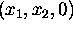. There is just one free parameter in the coordinates of an ideal point because the scale of a homogeneous vector is arbitrary - thus the set of all ideal points on the projective plane constitutes a line, called the ideal line. In the same way, the ideal points of projective 3-space have the form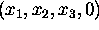and constitute a plane called the ideal plane, and so on for projective spaces of any dimension.

The image of an ideal point under a projectivity is called a vanishing point, the image of an ideal line is called a vanishing line, and so on. A familiar example of the formation of a vanishing point is shown in Figure 4. A camera with focal point C views a scene in which there are a pair of parallel lines M and N. Lines M and N appear in the image as converging lines m and n which meet at the vanishing point V. An important feature which is discussed below is that the line CV is parallel to lines M and N.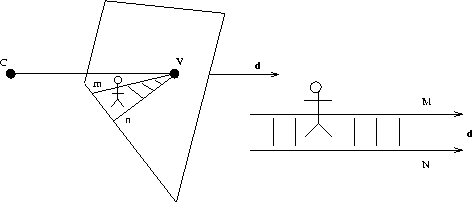Figure: The camera centre is at C. The parallel lines M and N in the scene give rise to converging lines m and n in the image which meet at the vanishing point V. The line CV is parallel to the lines M and N, with direction vector d.

An algebraic analysis of this configuration would describe the following - lines M and N are parallel and so meet at infinity at an ideal point; the image of M and N meeting at the ideal point is m and n meeting at the vanishing point V (the image of the ideal point is V) - note that concurrency is preserved under a projective transformation, so concurrency at the ideal point in the world is reflected in concurrency at V in the image; finally, the ray CV must itself be incident with the ideal point because this is perspective projection, and hence the ray CV is parallel to lines M and N in the scene. The analysis would involve some complication, because lines are more difficult to manipulate in projective 3-space than they are on the projective plane. In fact, an algebraic analysis is unnecessary to gain a reasonable understanding of the physical configuration, which can be obtained instead by a straightforward geometric argument as below.Figure: Two planes constructed in the way described in the text. By the arguments in the text, C and V are both on the line of intersection of the two planes, and the line of intersection has direction d (see Figure 4). Thus, CV is parallel to the lines M and N in the scene in Figure 4.

Referring again to Figure 4, consider a planethrough C and line M, and a plane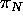through C and line N. We now show that the line of intersection of the two planes has the same direction d as lines M and N in the scene, and that CV coincides with the line of intersection of the two planes i.e. the line CV is parallel to the lines in the scene. See Figure 5.

First note that the line of intersection of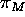andhas direction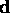because both planesandhaveas a basis vector. Now note thatintersects the image plane along m, and similarlyintersects the image plane along n. Thus, the line of intersection ofandpasses through the point of intersection of m and n which is the vanishing point V. The line of intersection ofandalso passes through C, because C is incident with both planes. Thus, CV coincides with the line of intersection ofand, and the argument is concluded.

The discussion can be extended to the imaging of a plane, as shown in Figure 6. A camera with focal point C views a tiled plane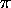. One set of parallel lines onis concurrent at the ideal point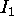, and the other set is concurrent at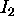. The line throughandis the ideal line (the horizon) of the plane. The image ofis the vanishing point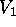, the image ofis the vanishing point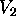, and the image of the ideal line is the vanishing line L. An important feature of this configuration, which can be demonstrated using similar arguments to those above, is that the normal of the plane defined by C and L is parallel to the normal of the plane- see Figure 6b.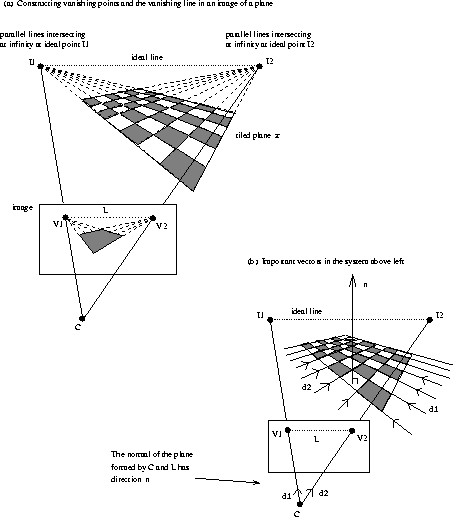Figure: (a) One set of parallel lines on the planeis concurrent at the ideal point, and the other set is concurrent at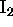. The line through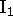andis the ideal line (the horizon) of the plane. The image ofis the vanishing point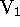, the image ofis the vanishing point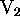, and the image of the ideal line is the vanishing line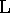. (b) Note that the plane formed by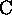and L has the same normal as the plane.